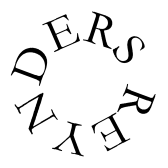# BINTIN (Ghana), Richard Darsie from Sean Feder - from Icon Tribe

`12/8      |:  .   .   *   .   .   *   .   .   *   .   .  :|`
`          _       _       _       _       _       _`
`Bottom    O   O   O   O   O   .   B   B   B   B   B   .`
`          _           _           _           _`
`Second    B   .   .   M   .   .   B   .   .   O   .   .`
`w/Stick   .   X   X   .   X   X   .   X   X   .   X   X`
`                  _       _               _       _`
`Third     .   .   B   .   O   .   .   .   B   .   O   .`
`w/Stick   X   .   .   X   .   .   X   .   .   X   .   .`
`          _       _       _       _       _       _`
`High      B   .   O   S   S   .   O   O   O   S   S   .`
`          _       _       _       _       _       _`
`Break     O   O   O  .    S   S   S   .   O   O   O   .`
`Bell      X   .   X   .   X   X   .   X   .   X   .   X`

`BOB         1 . . . 2 . . . 3 . . . 4 . . . 1 . . . 2 . . . 3 . . . 4 . . .`
`Djembe 1     b   t   b   t   b   s   s   s   b   t   b   t   b   s   s   s`
`Djembe 2     t   t   b   .   t   t   b   .   t   t   b   .   s   s   b   .`
`cont'd.     s   s   b   .   s   s   b   .   (back to beginning)`
`Djembe 3     b   t   t   b   s   s   b   t   t   b   s   s   b   t   t   etc.`

We like to have parts 1 and 2 play, say eight measures or so, then 1 and 3, then 2 and 3, and finally all three parts together.

The breaks: Opening and closing:

`      t   t   t   .   t   t   t   .   t   t   t   .   sf   .   sf`
`      t   t   t   .   t   t   t   .   t   t   t   .   sf   .   .`

Internal breaks: Call (played by drummer on the Djembe 2 part)

`                                                      sf   .   sf   .`

Response by all djembes:

`      t   t   t   .   t   t   t   .   t   t   t   .   sf   .   sf`

`Sangban      x      (x)      x      (x)      x       x       x        x`
`             O               O               O`
`stroke on the final 4 count can be doubled, as in                               x x`
`Kenkeni      x       x       x       x       x       x       x        x`
`                     O               O              (o)               O`